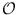Next: Topological Sort (flexible) ( Up: Graphs and Iterators Previous: Breadth First Search (flexible)   Contents   Index

# Depth First Search (flexible) ( GIT_DFS )

Definition

An instance algorithm of class GIT_DFS< OutAdjIt, Stacktype, Mark > is an implementation of an algorithm that traverses a graph in a depth first order. The stack used for the search must be provided by the caller and contains the source(s) of the search.

• If the stack is only modified by pushing the iterator representing the source node onto the stack, a normal depth first search beginning at the node of the graph is performed.

• It is possible to initialize the stack with several iterators that represent different roots of depth first trees.

• By modifying the stack while running the algorithm the behaviour of the algorithm can be changed.

• After the algorithm performed a depth first search, one may push another iterator onto the stack to restart the algorithm.

A next step may return a state which describes the last action. There are the following three possibilities:

1. dfs_shrink: an adjacency iterator was popped from the stack, i.e. the treewalk returns in root-direction
2. dfs_leaf: same as dfs_shrink, but a leaf occured
3. dfs_grow_depth: a new adjacency iterator was appended to the stack because it was detected as not seen before, i.e. the treewalk goes in depth-direction
4. dfs_grow_breadth: the former current adjacency iterator was replaced by the successor iterator, i.e. the treewalk goes in breadth-direction

Iterator version: There is an iterator version of this algorithm: DFS_It. Usage is similar to that of node iterators without the ability to go backward in the sequence.

#include < LEDA/graph/graph_iterator.h >

Creation

 GIT_DFS< OutAdjIt, Stacktype, Mark > algorithm(const Stacktype& st, Mark& ma) creates an instance algorithm of this class bound to the stack st and data accessor ma.

Preconditions:

• Stacktype is a stack parameterized with items of type OutAdjIt.
• st contains the sources of the traversal (for each source node an adjacency iterator referring to it) and
• ma is a data accessor that provides read and write access to a boolean value for each node (accessed through iterators). This value is assumed to be freely usable by algorithm.

 GIT_DFS< OutAdjIt, Stacktype, Mark > algorithm(const Stacktype& st, Mark& ma, const OutAdjIt& ai) creates an instance algorithm of this class bound to the stack st, data accessor ma and the adjacency iterator ai representing the source node of the depth first traversal.

Operations

 void algorithm.next_unseen() Performs one iteration of the core loop of the algorithm for one unseen node of the graph. dfs_return algorithm.next() Performs one iteration of the core loop of the algorithm. OutAdjIt algorithm.current() returns the current'' iterator. void algorithm.finish_algo() executes the algorithm until finished() is true, i.e. exactly if the stack is empty. bool algorithm.finished() returns true if the internal stack is empty. void algorithm.init(OutAdjIt s) initializes the internal stack with s. Stacktype& algorithm.get_stack() gives direct access to internal stack.

Implementation

Each operation requires constant time. Therefore, a normal depth-first search needs(m + n) time.Next: Topological Sort (flexible) ( Up: Graphs and Iterators Previous: Breadth First Search (flexible)   Contents   Index